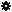﻿ Object Model: EdgeCollection.GrowNeighborEdges

EdgeCollection.GrowNeighborEdges

## EdgeCollection.GrowNeighborEdgesv3.0

### Description

Returns a collection of Edge objects for all edges that are adjacent to each point of each Edge in this collection, within a given distance.

### C# Syntax

 ```EdgeCollection EdgeCollection.GrowNeighborEdges( Int32 in_lDistance ); ```

### Scripting Syntax

 ```oReturn = EdgeCollection.GrowNeighborEdges( [Distance] ); ```

### Parameters

Parameter Type Description
Distance Integer Distance is an integer value representing the degree of neighborhood (eg: degree=2 for an edge means its adjacent edges plus the adjacent edges of the adjacent edges).

Default Value: 1

### Examples

#### VBScript Example

 ```set oCube = ActiveSceneRoot.AddGeometry( "Cube", "MeshSurface" ) set oGeometry = oCube.ActivePrimitive.Geometry set oEdges = oGeometry.Polygons(0).Edges set oNeighborEdges = oEdges.GrowNeighborEdges str = "Edges around the edges of the polygon(0) are :" for each n in oNeighborEdges str = str & " " & n.index next logmessage str ```